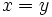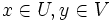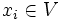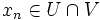# Hausdorff implies US

Jump to: navigation, search
This article gives the statement and possibly, proof, of an implication relation between two topological space properties. That is, it states that every topological space satisfying the first topological space property (i.e., Hausdorff space) must also satisfy the second topological space property (i.e., US-space)
View all topological space property implications | View all topological space property non-implications
Get more facts about Hausdorff space|Get more facts about US-space

## Statement

Any Hausdorff space is a US-space: every convergent sequence has a unique limit.

## Proof

Given: A Hausdorff space$X$. A convergent sequence$x_1, x_2, \dots$ with limit$x$ and limit$y$.

To prove:$x = y$.

Proof: Suppose$x \ne y$. Then, by Hausdorffness of$X$, there exist disjoint open subsets$U$ and$V$ such that$x \in U, y \in V$.

The definition of limit tells us that for all but finitely many$i$,$x_i \in U$, and for all but finitely many$i$,$x_i \in V$. Hence, there exists a value of$n$ for which$x_n \in U \cap V$, which is a contradiction since$U$ and$V$ are disjoint. This completes the contradiction, and hence the proof.